# Algebra II : Logarithms with Exponents

## Example Questions

2 Next →

### Example Question #61 : Simplifying Logarithms

Use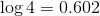and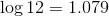Evaluate: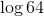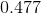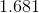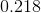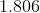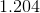Explanation:

Since the question gives,andTo evaluatemanipulate the expression to use what is given.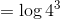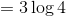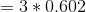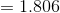### Example Question #62 : Simplifying Logarithms

Simplify: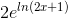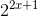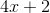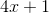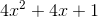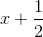Explanation:

According to log rules, when an exponential is raised to the power of a logarithm, the exponential and log will cancel out, leaving only the power.

Simplify the given expression.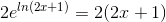Distribute the integer to both terms of the binomial.

The answer is:### Example Question #63 : Simplifying Logarithms

Simplify: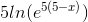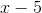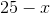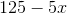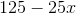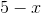Explanation:

The natural log has a default base of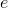.

This means that the expression written can also be: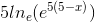Recall the log property that: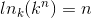This would eliminate both the natural log and the base, leaving only the exponent.

The natural log and the basewill be eliminated.

The expression will simplify to: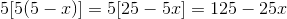The answer is:### Example Question #71 : Simplifying Logarithms

Simplify: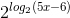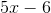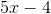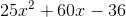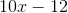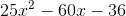Explanation:

The log property need to solve this problem is: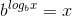The base and the log of the base are similar.  They will both cancel and leave just the quantity of log based two.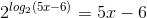The answer is:### Example Question #72 : Simplifying Logarithms

Solve: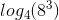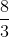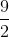Explanation:

Rewrite the log so that the terms are in a fraction.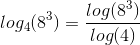Both terms can now be rewritten in base two.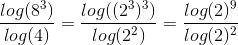The exponents can be moved to the front as coefficients.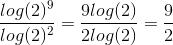The answer is:### Example Question #73 : Simplifying Logarithms

Which statement is true of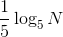for all positive values of?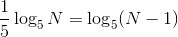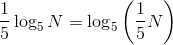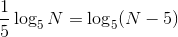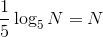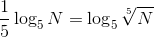Explanation:

By the Logarithm of a Power Property, for all real, all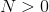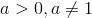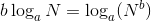Setting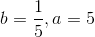, the above becomes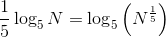Since, for any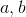for which the expressions are defined,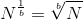,

setting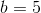, th equation becomes.

### Example Question #74 : Simplifying Logarithms

Which statement is true of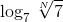for all integers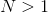?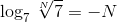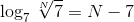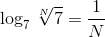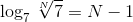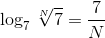Explanation:

Due to the following relationship: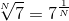; therefore, the expressioncan be rewritten as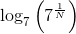By definition,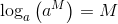.

Set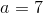and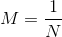, and the equation above can be rewritten as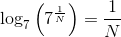,

or, substituting back,2 Next →

### All Algebra II Resources